## Artigo

•Citado por SciELO
•Acessos

•Citado por Google
•Similares em SciELO
•Similares em Google

## versão impressa ISSN 1692-3324

### Rev. ing. univ. Medellín vol.16 no.30 Medellín jan./jun. 2017

#### https://doi.org/10.22395/rium.v16n30a4

ARTÍCULOS

Calculation and Prediction of Fugacity and Activity Coefficients in Binary Mixtures, using a Self-Regulated Fretwidth Harmony Search Algorithm*

Cálculo y predicción de coeficientes de fugacidad y actividad en mezclas binarias mediante el algoritmo de búsqueda armónica con ancho de banda autoajustable

1Electrical engineer, Ph.D. (c). Universidad Industrial de Santander, Escuela de Ingenierías Eléctrica, Electrónica y de Telecomunicaciones. Bucaramanga, Colombia. Carrera 27 calle 9. Office LP-215. Tel.: 634400 Ext: 2954. E-mail: cristian.jimenez@correo.uis.edu.co.

2Mechatronics engineer, Ph.D. Universidad Industrial de Santander, Escuela de Ingenierías Eléctrica, Electrónica y de Telecomunicaciones. Bucaramanga, Colombia. Carrera 27 calle 9. Office LP-215. Tel.: 634400 Ext: 1295. E-mail: ivan.amaya2@correo.uis.edu.co. Current affiliation: Postdoc researcher, Instituto Tecnológico y de Estudios Superiores de Monterrey, National School of Engineering and Sciences, Research Group with Strategic Focus in Intelligent Systems. E-mail: iamaya2@itesm.mx.

3Chemical engineer, Ph.D. Professor, Universidad Industrial de Santander, Escuela de Ingenierías Eléctrica, Electrónica y de Telecomunicaciones. Bucaramanga, Colombia. Carrera 27 calle 9. Office AT-108. Tel.: 634400 Ext: 2954. E-mail: crcorrea@uis.edu.co.

Abstract

This research article proposes the use of a novel variant of the HS algorithm (harmony search), i.e. SFHS (self-regulated fret width harmony search algorithm), for calculating and predicting fugacity and activity coefficients in binary mixtures. Parameter selection was carried out based on preliminary results with different standard test functions. Different previously reported systems were selected, at 25 ° C and 40 ° C, and at low and moderate pressure levels. Moreover, two solutes were selected: carbon dioxide and ethane. Different solvents, both polar and nonpolar, were selected with comparative purposes. Activity and fugacity coefficients were calculated using the Redlich-Kwong state equation and Lewis rule, along with the sfhs algorithm, assuming both solutes in vapor phase. Consistency of the activity coefficients was analyzed by the Redlich-Kister strategy. Results were very close to those found experimentally by other authors, and most of them did not differ in more than one percentage unit.

Key words: fugacity coefficient; activity coefficient; binary mixtures; thermodynamic equilibrium; global optimization algorithms.

Resumen

Palabras clave: coeficiente de fugacidad; coeficiente de actividad; mezclas binarias; equilibrio termodinámico; algoritmos de optimización global

1. INTRODUCTION AND FUNDAMENTALS

Knowing the thermodynamic properties of mixtures and pure components is of paramount importance. Technical literature is prolific in reporting experimental data of properties such as activity and fugacity coefficients, as well as of other physicochemical parameters. Binary mixtures are particularly interesting, not only because of their wide industrial use, but also because they have been historically useful as models, with a relative ease for evaluating thermodynamic properties of a given component composition and nature. Likewise, as years have gone by and technology has improved, dependence on experimentation has diminished (though it has not been abandoned completely), trying to improve the prediction capability of theoretical models, or, even, of realistic and flexible semi-empiric ones.

On the other hand, accurate analysis of experimental data for modeling vle requires consistent numerical schemes, essential to have several alternatives for solving parameter estimation problems at hand. Traditional methods are easily trapped in local optima and do not guarantee convergence at all. In this sense, it is highly recommended to identify and study suitable approaches, such as the one used in the present work. As reported in the technical literature, the non-linear parameter estimation in vle modeling can be achieved either by solving a system of non-linear equations, a conventional method, or by direct optimization of the objective function 1.

Different components have been extensively studied at low and high pressures (including the critical region), mainly on binary mixtures. This list includes carbon dioxide, ethane, acetone, benzene, methanol, hexane, ethyl ether, and methyl acetate, amongst others 2-4. Throughout this study, we assume that binary mixtures are subject to near atmospheric pressure. Hence, it can be safely assumed that the properties of liquid phase are unaffected by pressure 2. However, this assumption does not apply to the gas phase, but its effect can be estimated with relative ease.

Classic literature shows the first approaches used for computer calculation of properties related to non-ideal, low pressure, liquid mixtures. They were composed of polar and non-polar substances, as well as of hydrogen links 3. Ohgaki et al., 4 achieved experimental values for two systems at moderate and high pressure levels, maintaining isothermal conditions: ethyl ether-carbon dioxide and methyl acetate-carbon dioxide. The authors determined activity and fugacity coefficients for the components of both mixtures. They reported that the activity coefficient was affected by the chemical nature of the solvent. In this sense, the activity coefficient of carbon dioxide (solute) was lower in the presence of methyl acetate, than in the presence of ethyl ether. This behavior was preserved throughout the whole range of studied mole fraction. Methyl acetate is slightly polar, and has two atoms that can donate electrons. Dioxide carbon, in this case, receives them. This means there is a strong interaction between both components, higher than between ethyl ether and carbon dioxide. Under low and moderate pressure levels, both binary systems exhibit a strong non-linear behavior in the fugacity coefficients. Still, they differ at high pressure levels, where the fugacity coefficient of the binary mixture ethyl ether-carbon dioxide becomes quite higher.

Afterwards, Ohgaki et al., 5 reported experimental data of the equilibria between liquid and vapor phases, under isothermal conditions, of three binary mixtures of carbon dioxide: methanol, n-hexane, and benzene. They considered moderate and high pressure levels, and reported activity and fugacity coefficients of each component in the mixtures through Lewis rule and Redlich-Kwong state equation for carbon dioxide in vapor phase. The authors demonstrated that the activity coefficient of carbon dioxide is considerably higher in the methanol-carbon dioxide mixture, and that the non-linearity of the liquid phase is due to the auto association present in methanol (as they suggested). This means that the link of hydrogen overcomes an eventual association with carbon dioxide. Likewise, experimental data shows a considerable deviation from non-linearity in the vapor phase of all binary mixtures. The highest one was exhibited by the n-hexane-carbon dioxide system, whilst the lowest one related to the methanol-carbon dioxide system.

Motivated to determine the effect of the chemical nature of the solute in the previously mentioned binary mixtures, Ohgaki et al., 6 swapped carbon dioxide with ethane, and reported results of the liquid vapor equilibria under isothermal conditions. They considered low to high pressure levels and a temperature of 25 °C. Furthermore, Redlich-Kwong state equation was used by the authors to calculate ethane fugacity coefficient. The activity coefficient of each solvent was calculated using a combination of Redlich-Kister equation with three parameters and the Gibbs-Duhem equation. The authors found, experimentally, that the highest activity coefficient of ethane for an infinite dilution is present in the methanol mixture, followed by mixtures considering acetone, methyl acetate, benzene, ethyl ether, and n-hexane. This order is altered if the solute is changed to dioxide carbon.

It would seem as if the self-association of solvents is the reason for a high activity coefficient of gas solute. Affinity between components cause an opposite effect. The authors highlight that activity coefficients of mixtures are higher if the solute is gas ethane, except for the n-hexane-ethane system. Likewise, they remark that non-linearity in liquid phase of the systems acetone-ethane, methyl acetate-ethane, and ethyl etherethane, are higher than when using carbon dioxide as a solute. Oppositely, the fugacity coefficient, as a non-ideality of the gas phase, were quite small for methanol-ethane systems and quite big for the n-hexane-ethane system. This tendency remained for carbon dioxide acting as a solute.

1.1 New Trends using Optimization Formulation

A brief literature survey regarding thermodynamic equilibria of binary mixtures, solved through modern optimization techniques, reveals an exponential growth that began during the 80’s (Figure 2), shows literature distribution by subject area. Most of what was found relates to engineering and chemistry, but areas such as computer science also contribute to this field 5-6.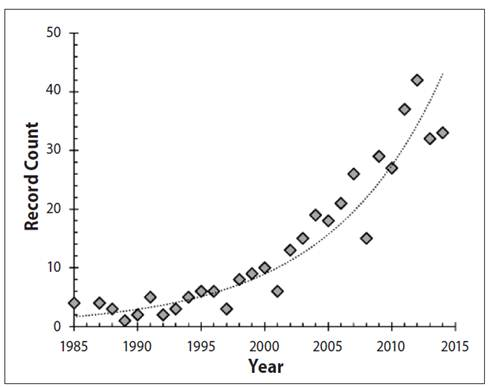Figure 1 Number of annual publications related to the implementation of optimization algorithms to describe the vapor liquid equilibrium.

More recent works deal with thermodynamic equilibria on reactive and nonreactive systems, through modern optimization techniques (1, 7-10). For example, S. Fateen and A. Bonilla reported on the use of swarm intelligence 7. They analyzed the performance of four algorithms: intelligent firefly algorithm (ifa), cuckoo search (cs), artificial bee colony (abc), and bat algorithm (ba). Their results were compared and the algorithms were ranked according to their trustworthiness and computational efficiency, using practical convergence criteria.

Sadeghi et al., 8 implemented a neural network model in tandem with RedlichKwong state equation and Pitzer expansion, and used it to determine the solubility of carbon dioxide in brine. The constants of the state equation were adjusted through genetic algorithms. Moreover, Lazzús 9 correlated liquid-vapor equilibria data at high-pressure levels for binary mixtures of supercritical fluids, and using a thermodynamic optimization model and a particle swarm algorithm. The thermodynamic model was based in the Peng-Robinson state equation, and it included the empirical Wong-Sandler mixing rules, as well as Van Laar model for excess free Gibbs energy.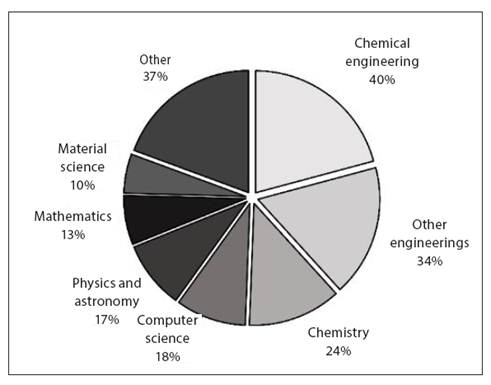Figure 2 Distribution of reports by subject area, regarding the literature analysis carried out.

In this article, we use a quick and convenient way of predicting fugacity and activity coefficients of some systems, previously reported in literature. As known, the chemical potential is a thermodynamic variable useful for describing phase equilibria. Nowadays, application of these criteria is supported by considering the fugacity, f , which replaces the chemical potential, μ, while lacking its drawbacks. Fugacity coefficient, φ, represents the relation between the system’s fugacity and pressure level. In the special case of an ideal gas, fugacity equals the system pressure, meaning that the fugacity coefficient equals unity 2.

Activity coefficient of a species in a solution represents the relation between the real fugacity and the fugacity corresponding to an ideal solution (calculated via Lewis-Randall rule). This under the same assumptions of temperature, pressure, and composition. According to the Lewis-Randall rule, the fugacity of component i in a mixture of ideal solution, is proportional to its mole fraction. Proportionality constant

is the fugacity of pure species i, i.e.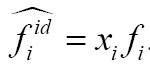. Activity coefficient is a comparative measure that determines how component i behaves within the solution ideally (2).

The current article now moves on to a brief description of the recently proposed sfhs algorithm. Then, it discusses the proposed methodology for calculating fugacity and activity coefficients in the selected binary samples, showing the main equations required for its determination. Afterwards, the most relevant results and simulations are presented, their meanings are analyzed. The paper wraps up with a discussion of the most significant conclusions.

2. MATERIALS AND METHODS

2.1 The Self-Regulated Fretwidth Harmony Search (sfhs) Algorithm

This algorithm is similar to the original hs approach (10), and it was inspired on the success of the abhs variants (11). Still, it is also possible to vary the Fretwidth (FW) with each iteration, based on the following three events:

2. If the best solution in HM is improved, look for good values around the current

3. After FWsat produces non-successful iterations, switch to an exponential decay.

The first event is quite clear, so we will not elaborate on it. Similarly, the third event is quite close to the one proposed in abhs, and thus we invite the reader to check (11) for an in-depth explanation. The remaining case (i.e., scenario two) is the core of our proposal and it is ruled by equation (1). Throughout this behavior, a random number uniformly distributed between zero and one (rFW) is multiplied by a constant (CFW), to stochastically adjust the fretwidth (FW) around the value that leads to improving the solution (Aj).

The latter (i.e., Aj) represents the midpoint for random generation of new fretwidths. This stochastic behavior is maintained until the third event is repeated. Graphically, this phenomenon is represented in Figure 3, assuming that a better solution is found at iterations 5, 24, and 27. The logic of the current proposal is summarized in Figure 4. It is worth noting that CFW is included in the equation as a mean of controlling the amplitude of the adjustment on Aj. However, we explore, in this work, the particular case of setting CFW = 1. This means that the new value of Aj will lay inside the interval [0.5A j, 1.5A j ].

It is important to comment on the feasible ranges for each one of the remaining parameters. The harmony memory size (hms) indicates how many solutions are stored and considered during the execution of the algorithm. The harmony memory considering rate (hmcr) and pitch adjusting rate (par) are values that must be located between zero and one, since they relate to the probability of selecting a given path in our proposed algorithm. Based on previously reported recommendations (12), we defined FWini = 0.5, FWmax = 2.0, FWmin = 10-25, FW = 1, 000, and SatHS= 10, 000. The remaining parameters, i.e. hms, par, and hmcr, were selected as will be discussed in the results section.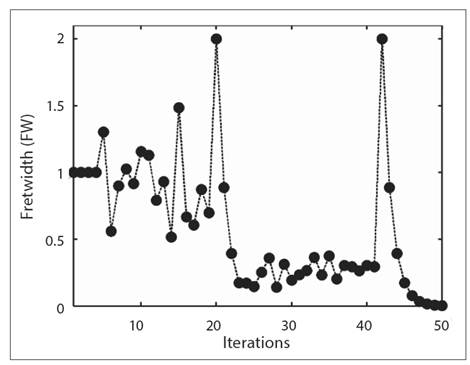Figure 3 Sample variation of FW as a function of the iterations, considering that a better answer is found at iterations 5, 24, and 27.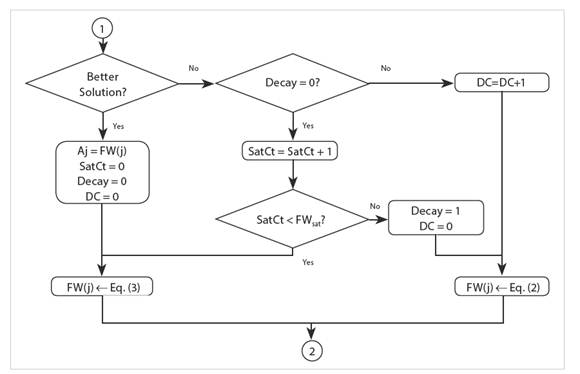Figure 4 Logic of the SFHS variant, where DC is the decay counter, j is the current iteration, Satct is the saturation counter, and FWsat is the saturation limit.

2.2 Methodology

The methodology applied takes advantage of a computational model for predicting fugacity and activity coefficients, through sfhs.

2.2.1 Input of experimental parameters

The first step requires feeding the algorithm with experimental data. In this sense, the following variables were taken from reported literature (2-4): system pressure and temperature; mole fraction of the mixture; components of the mixture; critical values of each component, and their acentric factor.

2.2.2 Domain definition for each function

One component of the mixture is selected (carbon dioxide was chosen and used throughout this study,). The mole fraction in liquid phase is split into 100 points. Both phases (i.e. liquid and gas) of the other component (methanol, hexane, benzene, ethyl ether, and methyl acetate) in thermodynamic equilibrium, are also split into 100 points.

2.2.3 Calculation of fugacity coefficient for component two (solute)

We start with the modified Redlich-Kwong state equation, i.e., where p: pressure (atm); T: temperature (K); v: specific molar volume of the system (m3/mol); R: the universal constant (atm m3/mol K); a(T) and b: pure component constants for this state equation.

The values of constants L and Y in the modified Redlich-Kwong state equation for a given substance, as shown in equation (3), can be determined knowing the critical properties and the experimental conditions as described in (17).

Furthermore, coefficient Λ can be calculated with the help of equation (4):

wi is the acentric factor for the solute. Now, considering those relations, the cubic state equation can be rewritten as a function of the compressibility factor, as depicted in equation (5),

This nonlinear equation can be solved to find three possible values for the compressibility factor Z. Out of these values, the highest one is chosen. This selection represents the physical situation with highest volume, i.e. the gas phase. After finding these parameters, fugacity coefficient for the solute can be obtained as follows. Using the definition of the fugacity coefficient as a function of the above parameters, we reach equation (6). Since the values of mole fraction related to carbon dioxide are close to unity, fugacity coefficient nears that of a pure substance.

2.2.4 Calculation of activity coefficient for component two (solute)

Because during thermodynamic equilibria the fugacity of vapor and liquid phases ( f v and f L respectively) are the same, the former then complies with equation (4) whilst the later complies with equation (5). Solving these two equations leads to equation (6a).

P: the system’s pressure in atmospheres; R: the universal gas constant; f2V: gas fugacity for component two; f2L: liquid fugacity for component two; φ2,pure: solute fugacity coefficient; x2: mole fractions of the liquid phase; γ 2 : the mole fractions of the gas phase; f2L(PO)3: liquid fugacity at reference pressure of 0 atm;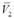: solute partial cm3mole-1 ; T: system temperature; γ2: solute activity coefficient. It can then be assumed that the partial mole volume of carbon dioxide,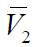, is close to the partial mole volume under infinite dilution, V2∞, and that it is independent of pressure. Likewise, fugacity of pure carbon dioxide was taken from literature, at reference pressure (i.e. at zero atmospheres) and at 25 °C (2). The sfhs algorithm was used to calculate the best values of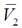and f 2 L( PO) .

2.2.5 Calculation of activity coefficient for component one (solvent)

Now, in order to determine activity coefficients, the Redlich-Kister equation was used, as shown in equations (7) and (8). Subtracting these equations leads to equation (9), where is the activity coefficient, x is the molar fraction and coefficients B, C, and, D are correlated through just one equation. This feature allows obtaining the activity coefficients of the component one, based on experimental data of the other component (for binary mixtures).

2.2.6 Calculation of fugacity coefficient for component one (solvent)

Based on the analog representation of the fugacity equality principle, equation (10) is derived for the solvent of the binary mixture. Here, it was assumed that the partial molar volume of the solvent,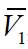, is close to the mole volume of pure solvent, V1, and independent of pressure. The sfhs algorithm was used to determine the best values ofand f 1 L(PO) .

x 1: mole fraction of the liquid phase for component one; y1: mole fraction of the and gas phase for component one; f 1 L(PO) : liquid fugacity at reference pressure of 0 atm;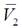:solvent partial molar volume given in m3mol -1; γ2: solvent activity coefficient.

2.2.7 The objective functions

Knowing the thermodynamic definitions for fugacity and activity coefficients, the following objective functions Fobj were assembled:

After solving these equations using the sfhs optimization algorithm, the following parameters were estimated: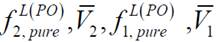, B,C and D. Additionally, these values lead to constructing all figures described in the next section.

3. RESULTS AND DISCUSSION

Before running the actual simulations, it was necessary to probe the ability and performance of the sfhs optimization algorithm. Striving to identify appropriate values for each required parameter, a tuning stage was carried out. Still, and considering space restrictions, we deemed more important to present all data regarding the particular application (i.e. calculation and prediction of fugacity and activity coefficients), since it is the main novelty of this manuscript. Thus, just an excerpt of tuning data is shown below.

3.1 Parameter Selection for sfhs

Different feasible ranges were considered, and tests were repeated 50 times in order to gather sufficient data. All tests were run with different seeds to favor randomness.

In the case of the harmony memory considering rate (hmcr), the study was restricted to 0.2, 0.5, and 0.9, whilst in the case of the pitch adjusting rate (par), the analysis hovered above 0.2, 0.5, and 0.8. The main reason for not considering higher par values was that it implied adjusting each solution almost every time, thus resembling a random walk algorithm. The total number of solutions considered at any given time (hms) was either five or ten.

Resulting data showed a clear effect due to hmcr and a somewhat smaller effect of par. In the first case (Figure 5), using a mid or low value implies increasing the number of iterations and, hence, the strategy’s convergence time. This effect can be so marked that the algorithm stops due to an excessive number of iterations. In the second case (Figure 6), the difference is harder to notice. Still, a high par reduces the number of required iterations and convergence time, especially at higher dimensions.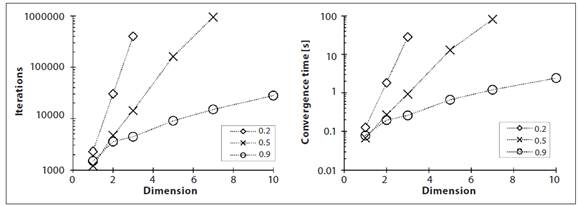Figure 5 Average number of iterations (left) and convergence time (right) after 50 runs considering the shifted Jong function and different values of HMCR. HMS = 5, PAR = 0.8.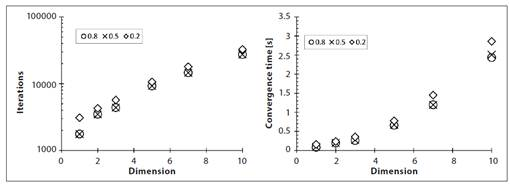Figure 6 Average number of iterations (left) and convergence time (right) after 50 runs considering the shifted Jong function and different values of PAR. HMS = 5, HMCR = 0.9.

The difference in performance when changing hms was also evident. As an example, consider the data shown in Figure 7, where the shifted Jong function is analyzed for different sizes while keeping hmcr and par at the best values found (i.e. 0.9 and 0.8, respectively). It becomes evident that increasing the memory size hinders sfhs performance. Thus, execution parameters for fugacity and activity tests were selected as hmcr= 0.9, par= 0.8, and hms= 5. The remaining parameters were set following literature recommendations, as discussed above (12).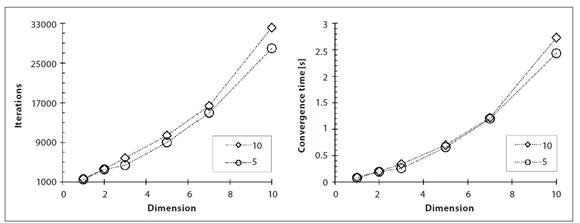Figure 7 Average number of iterations (left) and convergence time (right) after 50 runs considering the shifted Jong function and different values of HMS. HMCR = 0.9, PAR = 0.8.

We now present some simulation results obtained by applying the proposed methodology to the selected binary systems.

3.2 System: Methanol CO2

Figure 8 shows data derived from simulations. The predictions given by the computational model are shown as a continuous line, whilst the reference values of activity and fugacity coefficients are denoted by circles. Temperature of the mixture was kept constant at 25 °C, and predicted, its reference values were agreed. The parameters of the mixture achieved through sfhs, and the reference data points, (2-3), are shown in Table 1.

Table 1 Thermodynamic parameters for the mixture methanol-CO2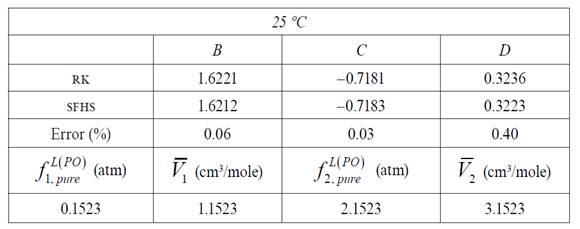Source: authors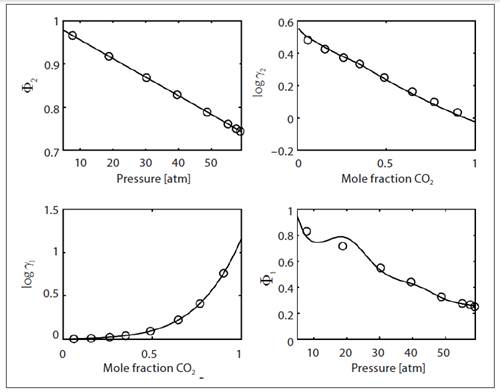Figure 8: Values of fugacity and activity coefficients for methanol (component one) and carbon dioxide (component two: solute) in the mixture, at a temperature of 25°C.

Likewise, Figure 9 shows that increasing the temperature of the mixture to 40

°C does not hinder the accuracy of the proposed approach. The parameters for this scenario are summarized in Table 2.

Table 2 Thermodynamic parameters for the mixture methanol-CO2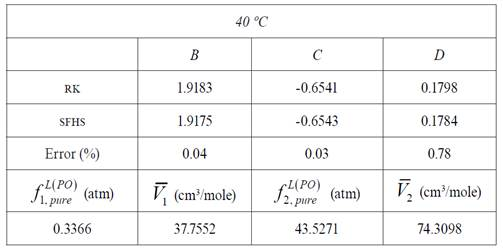Source: authors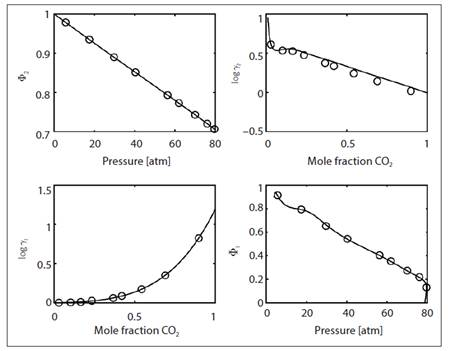Figure 9 Values of fugacity and activity coefficients for methanol (component one) and carbon dioxide (component two: solute) in the mixture at a temperature of 40°C.

3.3 System: Hexane CO2

Afterwards, the Hexane CO2 binary mixture at a constant temperature of 25 °C was selected. Simulation results are shown in Figure 10, based on the parameters shown in table 3. The agreement between simulated and experimental results is remarkable. The fugacity coefficient curve of the CO2 vapor phase was found using the Redlich-Kwong state equation, assuming it is in pure state.

Table 3 Thermodynamic parameters for the mixture hexane-CO2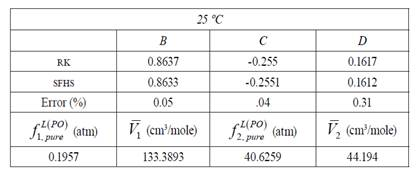Source: authors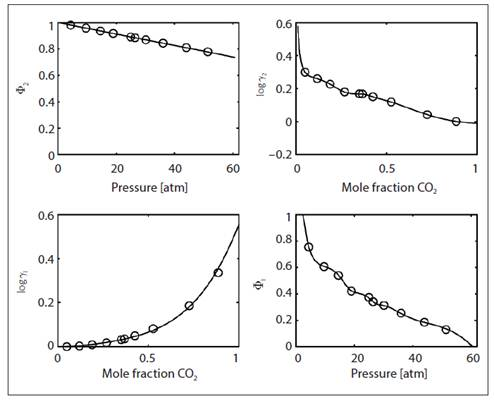Figure 10 Values of fugacity and activity coefficients for hexane (component one) and carbon dioxide (component two: solute) in the mixture at a temperature of 25°C.

Figure 11 also shows an excellent agreement between simulations and experiments for the same mixture, but this time at 40 °C. The main results of the simulation are summarized in Table 4. Again, constants defining activity coefficients of each component are equivalent to those previously reported in literature, with error values below 1% (13).

Table 4 Thermodynamic parameters for the mixture hexane-CO2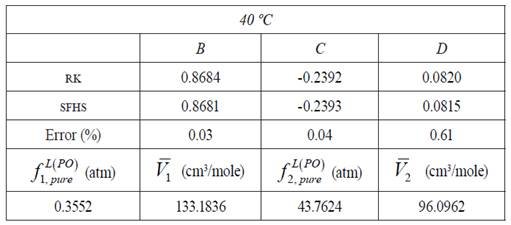Source: authors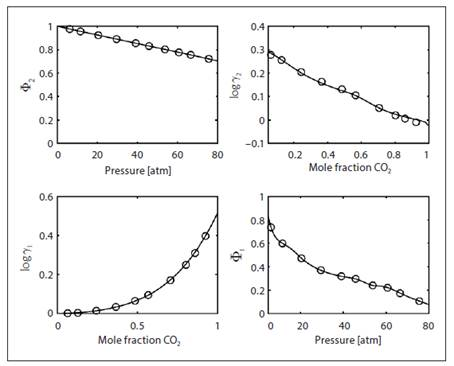Figure 11 Values of fugacity and activity coefficients for hexane (component one) and carbon dioxide (component two: solute) in the mixture at a temperature of 40°C.

3.4 Other systems with carbon dioxide as a solute

A third approach considered was the Benzene CO2 mixture. In this case, fugacity and activity coefficients behave as shown in Figure 12 for a temperature of 25 °C, and as shown in Figure 13 for a temperature of 40 °C. The respective thermodynamic properties, given by simulations, are compiled in Tables 5 and 6, respectively. Changing the solvent to ethyl ether does not hinder the efficiency of the proposed approach, as can be seen in Figure 14 and 15 for 25 °C and 40 °C, respectively. Likewise, using methyl acetate as a solvent allows good results, as seen in Figures 16 and 17 for the same temperature levels. Thermodynamic properties of these tests are shown in Tables 7 and 8.

Table 5 Thermodynamic parameters for the mixture benzene-CO2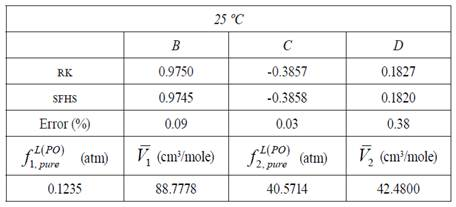Source: authors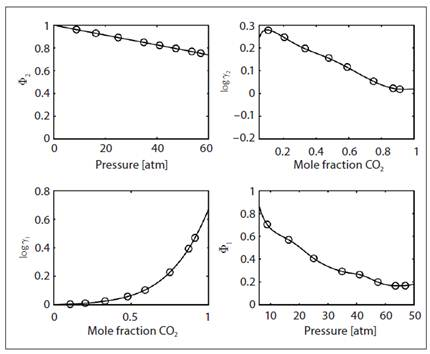Figure 12 Values of fugacity and activity coefficients for benzene (component one) and carbon dioxide (component two: solute) in the mixture at a temperature of 25°C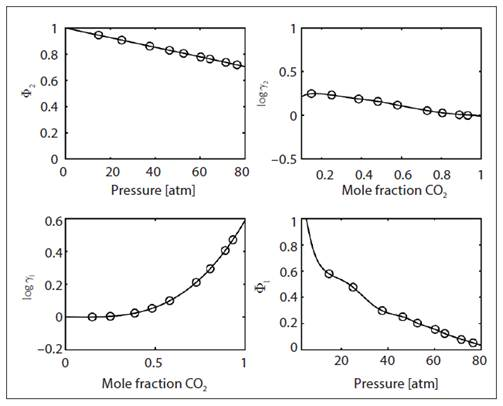Figure 13 Values of fugacity and activity coefficients for benzene (component ne) and carbon dioxide (component two: solute) in the mixture at a temperature of 40°C

Table 6 Thermodynamic parameters for the mixture benzene-CO2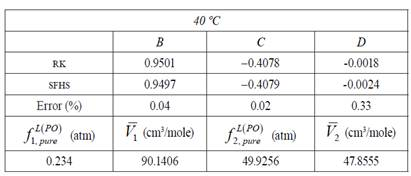Source: authors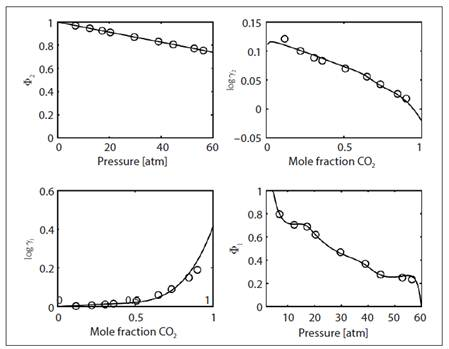Figure 14 Values of fugacity and activity coefficients for ethyl ether (component one) and carbon dioxide (component two: solute) in the mixture at a temperature of 25°C. .

Table 7 Thermodynamic parameters for the mixture ethyl ether-CO2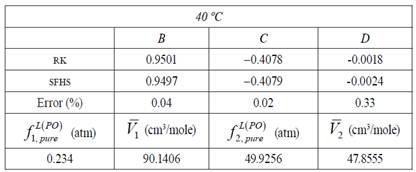Source: authors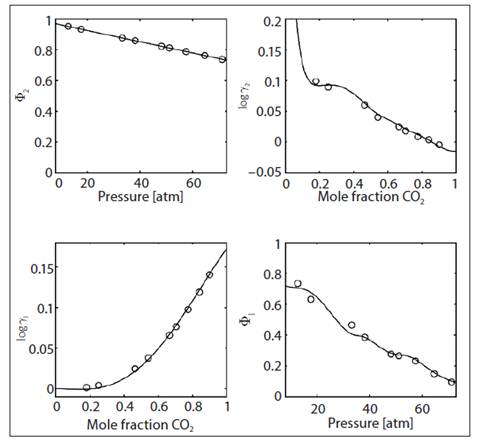Figure 15 Values of fugacity and activity coefficients for ethyl ether (component one) and carbon dioxide (component two: solute) in the mixture at a temperature of 40°C.

Table 8 Thermodynamic parameters for the mixture ethyl ether-CO2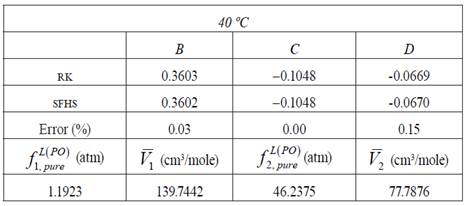Source: authors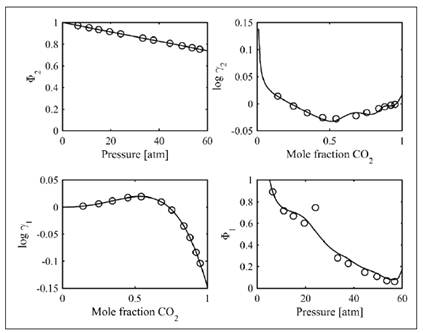Figure 16 Values of fugacity and activity coefficients for methyl acetate (component one) and carbon dioxide (component two: solute) in the mixture at a temperature of 25°C.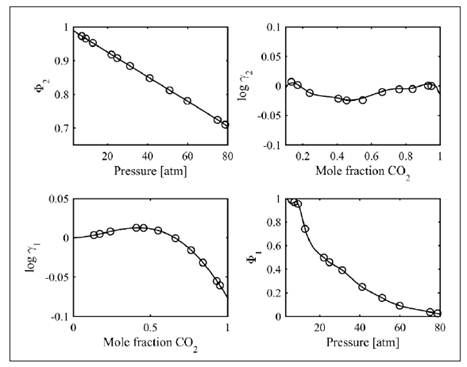Figure 17 Values of fugacity and activity coefficients for methyl acetate (component one) and carbon dioxide (component two: solute) in the mixture at a temperature of 40°C.

3.5 BINARY MIXTURES WITH ETHANE AS SOLUTE

In order to establish the reproducibility of the proposed numerical calculation, we calculates the fugacity and activity coefficients for several new binary mixtures where the solute was ethane. In this case, we obtained the results presented in Figures 18 to 22. As can be seen, the results for these binary systems also agree quite well with those reported in the literature, showing that this numerical approach is valid, at least for the systems analyzed.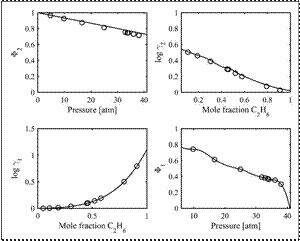Figure 18 Values of fugacity and activity coefficients for methyl acetate (component one) and ethane (component two: solute) in the mixture at a temperature of 25°C.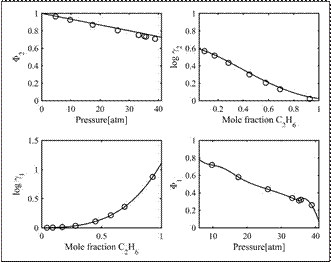Figure 19 Values of fugacity and activity coefficients for acetone (component one) and ethane (component two: solute) in the mixture at a temperature of 25°C.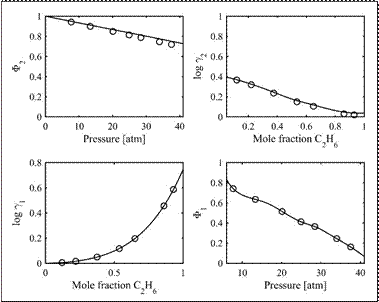Figure 20 Values of fugacity and activity coefficients for benzene (component one) and ethane (component two: solute) in the mixture at a temperature of 25°C.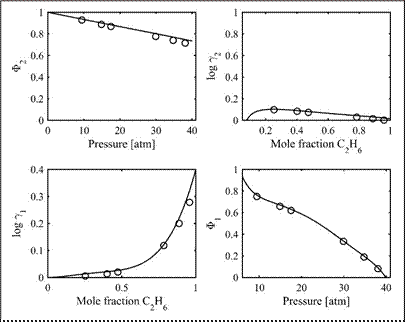Figure 21 Values of fugacity and activity coefficients for ethyl ether (component one)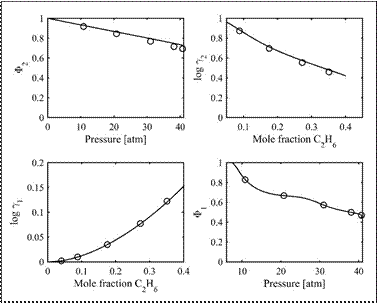Figure 22 Values of fugacity and activity coefficients for methanol (component one) and ethane (component two: solute) in the mixture at a temperature of 25°C.

3.6 ACTIVITY COEFFICIENTS CONSISTENCY

After having calculated all activity coefficients, we checked their coherence. Due to space restrictions, only two randomly selected examples are reported here. The method involves calculating the derivative of the free excess energy with respect to the composition, at constant temperature and pressure. Then, the logarithmic relationship is calculated, so that if they are consistent, equation (14) must be satisfied.

3.6.1 System: methanol CO2 at 25 ºC

Figure 23 shows the logarithmic change of activity coefficients for this mixture, as a function of its composition. As seen, the total area under the curve is close to zero (7.3 x 10-3). The Riemann sum method was used for its calculation by setting a value on the abscissa step equal to 0.01. It is worth noting that the function has maximum amplitude of 3.88 units. So, we can say with high confidence that the values of the activity coefficients are consistent for this binary mixture.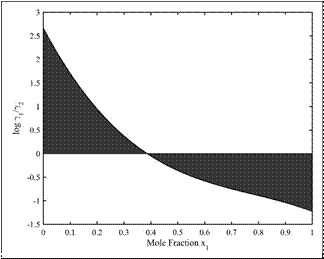Figure 23 Ratio of the activity coefficients in the system methanol CO2 at a temperature of 25°C.

3.6.2 System: benzene ethane at 25 °C

For this binary system, Figure 24 shows the logarithmic change of activity coefficients as a function of its composition. Again, the total area is close to zero. Based on the same numerical method as before, this integral yields a value of 3.5 x 10-3. This value was obtained with a step in the coordinate of the mole fraction of hexane to 0.01 units. It is noted that the function has maximum amplitude of 2.74 units. Similarly, it follows that activity coefficients are consistent and the assumptions made in their calculations are acceptable within the permissible error. Thus, calculation of activity coefficients by the sfhs algorithm constitutes a viable alternative tool for binary mixtures (14).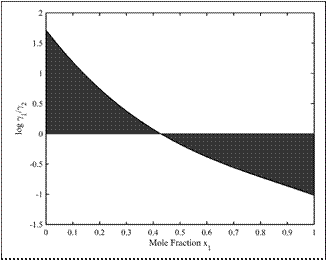Figure 24 Ratio of the activity coefficients in the system benzene ethane, at a temperature of 25°C.

CONCLUSIONS

Throughout this article, it was demonstrated that fugacity and activity coefficients can be obtained via the sfhs optimization algorithm. By comparing our data against previously reported experimental results for different binary systems, we observed that our results are accurate within the error margin. Hence, the proposed computational strategy is valid and it can be easily implemented. Similarly, the values of activity coefficients were consistent, as was confirmed using the Redlich-Kister approach, for the two randomly selected examples.

ACKNOWLEDGMENTS

The authors gratefully acknowledge the financial support of Universidad Industrial de Santander and Colciencias.

REFERENCES

1. A. Bonilla-Petriciolet, “On the capabilities and limitations of harmony search for parameter estimation in vapor-liquid equilibrium modeling,” Fluid Phase Equilibria, no. 332, pp. 7-20, 2012. [ Links ]

2. J. M. Smith, H. C. Van Ness and M. M. Abbott, Introduction to Chemical Engineering Thermodynamics, México: Mc Graw Hill, 1995. [ Links ]

3. J. M. Prausnitz, Computer Calculations For High Pressure Vapor Liquid Equilibria, Prentice Hall International Series, 1968. [ Links ]

4. K. Ohgaki, F. Sano and T. Katayama, “Isothermal Vapor-Liquid Equilibrium Data for Binary Systems Containing Ethane at High Pressures,” Journal of Chemical and Engineering Data, vol. 21, no. 1, pp. 55-58, 1976. [ Links ]

5. K. Ohgaki and T. Katayama, “Isothermal Vapor-Liquid Equilibria for Systems Ethil EtherCarbon Dioxide and Methil Acetate-Carbon Dioxide at High Pressures,” Journal of Chemical and Engineering Data, vol. 20, no. 3, 1975. [ Links ]

6. K. Ohgaki and T. Katayama, “Isothermal Vapor-Liquid Equilibrium Data for Binaryt Systems Containing Carbon Dioxide at High Pressures: Methanol-Carbon Dioxide, n-Hexane-Carbon Dioxid, and Benzene-Carbon Dioxide Systems,” Journal of Chemical and Engineering Data, vol. 21, no. 1, 1976. [ Links ]

7. S. E. Fateen and A. Bonilla, “On the performance of swarm intelligence optimization algorithms for phase stability and liquid-liquid and vapor-liquid equilibrium calculations,” Periodica Polytechnica Chemjical Engineering, vol. 59, no. 3, pp. 186-200, 2015. [ Links ]

8. M Sadeghi, H Salami, V. Taghikhani and M Robert, “A comprehensive study on CO2 solubility in brine: Thermodynamic-based and neural network modeling,” Fluid Phase Equilibria, vol. 403, pp. 153-159, 2015. [ Links ]

9. J. A. Lazzús, “Optimization of high-pressure vapor-liquid equilibrium modelling of binary mixtures (supercritical fluid + ionic liquid) by particle swarm algorithm,” University of Kragujevac, Faculty of Science, vol. 73, no. 3, pp. 663-688, 2015. [ Links ]

10. Z. Geem, J. Kim and G. Loganathan, “A New Heuristic Optimization Algorithm: Harmony Search,” Simulation, no. 76, pp. 60-68, 2001. [ Links ]

11. J. Contreras, I. Amaya and R. Correa, “An improved variant of the conventional Harmony Search algorithm,” Appl. Math. Comput ., no. 227, pp. 824-830, 2014. [ Links ]

12. I. Amaya, J. Cruz and R. Correa, “Harmoy Search algorithm: a variant with Self-regulated Fretwidth,” Appl. Math. Comput ., no. 266, pp. 1127-1152, 2015. [ Links ]

13. O. A. Hougen, K. M. Watson and R. A. Ragatz, Chemical Process Principles Part II. Thermodynamics, New York: John Wiley & Sons, Inc., 2005. [ Links ]

14. J. López, V. Trejos and C. Cardona, “Objetive functions analysis in the minimization of binary VLE data for asymmetric mixtures at high pressures,” Fluid Phase Equilibria, no. 248, pp. 147-157, 2006. [ Links ]

15. D.-Y. Peng and D. B. Robinson, “A New Two Constant Equation of State,” Journal of Industrial and Engineering Chemistry, vol. 15, no. 1, 1976. [ Links ]

16. G. Soave, “Equilibrium constants from a modified Redlich-Kwong equation of state,” Chemical Engineering Science, vol. 27, no. 6, pp. 1197-1203, 1971. [ Links ]

17. L. N. Canjar and F. S. Manning, Thermodynamic Properties and Reduced Correlations for Gases, Houston: Gulf Publishing Company, 1967. [ Links ]

18. J. López, V. Trejos and C. Cardona, “Parameters estimation and VLE calculation in asymmetric binary mixtures containing carbon dioxide + n alkanols,” Fluid Phase Equilibria, no. 275, pp. 1-7, 2009. [ Links ]

19. A. Bharti, Prerna and T. Banerjee, “Applicability of Cuckoo Search Algorithm for the Prediction of Multicomponent Liquid-Liquid Equilibria for Imidazolium and Phosphonium Based Ionic Liquids,” Industrial and Engineering Chemistry Research, vol. 54, no. 49, pp. 12393-12407, 2015. [ Links ]

20. J. Fernández-Vargas, A. Bonilla-Petriciolet and J. Segovia-Hernández, “An improved ant colony optimization method and its application for the thermodynamic modeling of phase equilibrium,” Fluid Phase Equilibria, no. 353, pp. 121-131, 2013. [ Links ]

21. V. Bhargava, S. Fateen and A. Bonilla-Petriciolet, “Cuckoo Search: A new nature-inspired optimization method for phase equilibrium calculations,” Fluid Phase Equilibria, no. 337, pp. 191-200, 2013. [ Links ]

22. A. Kabouche, A. Boultif, A. Abidi and N. Gherraf, “Interaction parameter estimation in liquid-liquid phase equilibrium modeling using stochastic and hybrid algorithms,” Fluid Phase Equilibria, no. 336, pp. 113-121, 2012. [ Links ]

1Article derived from the research proposal Thermodynamics of the precipitation of waxes under the influence of electric fields, developed between February 2013 and November 2015. This work was supported by the Uni- versidad Industrial de Santander, under the PhD program in engineering with the internal code 308.

Received: August 24, 2015; Accepted: March 18, 2016This is an open-access article distributed under the terms of the Creative Commons Attribution License